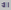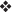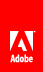# Using decimal and numeric fields

Decimal fields are very similar to numeric fields. The following table describes the differences between decimal and numeric fields.

Field

Description

Decimal field

Displays numbers in decimal format.

You can set the maximum number of leading and trailing digits.

You can set a display pattern to restrict the formatted value with the leading and trailing digits.

You cannot specify a data format for a decimal field; it is always stored as decimal.

By default, the formatted value includes two digits following the radix character.

Numeric fields

Displays numbers in float or integer format.

You cannot set the maximum number of leading and trailing digits.

You can set a data pattern and data format (either float or integer).

The formatted value includes only two digits following the radix character.

Use the decimal field in the following cases:

• If you want to set the number of leading or trailing digits

• If you want the precision of decimal or integer data to be shown exactly as it is entered (by ensuring the Limit Trailing Digits option is deselected) without any truncating

For example, a field called Qty is located within a repeating subform. In the first instance of the subform, the Qty field may have the value 3, and in the second instance of the subform, the Qty field may have the value 3.123:

• If the Limit Trailing Digits option is set to a maximum of 2, the first number is stored as 3 (because trailing 0s are removed) and the second number is stored as 3.12 (because the number is truncated).

• If the Limit Trailing Digits option is deselected, both numbers are stored exactly as they are entered (the first number is stored as 3 and the second number is stored as 3.123).

To learn more about the options for decimal fields, see the section on numeric fields because many of the options are identical.

Note: To use the decimal field object in an interactive form, you must have Adobe Reader 6.0.3 or later.

If you want to support the collection or display of float or integer data, including currency, add a numeric field to the form. The value in a numeric field can be formatted and displayed according to predefined patterns and according to the locale specified for the field.

After you add a numeric field to the form design, you can edit the caption text and manipulate the object’s properties in the Field, Value, and Binding tabs of the Object palette. You can define these properties:

Numeric fields support scripting and calculations. If a user is to supply data, you can define whether the input is recommended or required, and you can set up messages to prompt users appropriately. All user input may be validated through scripting.

Note: Numeric fields have a maximum user-entered or calculated value of 2,147,483,647. This number is the largest number that can fit into a 32-bit signed integer on a computer.

## To define a radix (decimal point) alignment for numeric values

1. In the Paragraph palette menu, select Edit Value.

2. Click Radix Alignment.

3. In the adjacent box, type the amount of space to create between the radix point and the right edge of the fillable area.

4. Press Enter.

Important: Acrobat 6.0.2 and Adobe Reader 6.0.2 do not support the radix alignment setting. If you intend to save the design as an Acrobat 6.0.2-compatible PDF form, do not use radix alignment. If you do, users cannot edit the values.

## To define the behavior of decimal or numeric fields

1. In the Object palette, click the Value tab and select one of these options from the Type list:

• To allow users to choose whether to enter data, select User Entered - Optional.

• To prompt users to enter data and make the field recommended, select User Entered - Recommended and type a custom message in the Empty Message box.

• To prompt users to enter data and make the field required, select User Entered - Required and type a custom message in the Empty Message box.

• To make the field read only and display a data value that is calculated and displayed through an attached script, select Calculated - Read Only. Users cannot edit the calculated value.

• To make the field editable and display a data value that is calculated and displayed through an attached script, select Calculated - User Can Override. Users can edit the value if the calculation script has been written to accept the input. If a user does edit the calculated value, the custom message you specify in the Override Message box appears.

• To make the field read only and display a data value that is merged or calculated and displayed at run time, select Read Only. Users cannot edit the value.

2. If the value is recommended or required, type a prompt in the Empty Message box.

3. If the value will be calculated, attach the calculation script to the object by using the Script Editor.

4. (Optional) If a calculated value can be overridden, type a message in the Override Message box.

## To define custom data-binding properties for decimal or numeric fields

Binding options enable you to build a form that captures data for enterprise infrastructures and/or use an external data source to populate a form at run time. Set data-binding properties in the Binding tab of the Object palette.

1. Select the field.

2. Enable the form to connect to the data source when the form is opened.

3. Bind the field to its corresponding data node. For information about how to bind objects to a data source, see Binding fields to a data source .

## To specify the data format for numeric fields

Numeric fields can save data in Float or Integer format. The default is Float.

By default, numeric data in a Decimal Field will only save a maximum of two digits after the decimal. Data beyond the second decimal place will be rounded.

1. In the Object palette, click the Binding tab.

2. Select the appropriate format from the Data Format list:

• To specify the number format as a three-part representation of a number that contains a radix character, select Float.

• To specify the number as any sequence of the digits 0 through 9, possibly preceded by a minus sign, select Integer.

## To limit the numbers entered in decimal and numeric fields

You can limit how many numbers may be entered in decimal and numeric fields by specifying that Designer use the horizontal length of the decimal or numeric field to determine how many numbers to allow.In the Object palette, click the Field tab and select Limit Length to Visible Area.

## To perform a calculation with decimal fields by using JavaScript

You can perform calculations with decimal fields by using FormCalc. However, if the Limit Trailing Digits option is deselected, and you want to use JavaScript to perform a calculation with decimal fields, you must use a script. To maintain a high degree of precision, Designer stores the value of decimal fields as a string. The script indicates to the decimal field that it is a number.

For example, you have three decimal fields in your form design. The first is named a , the second b , and the third Total . You want to perform a calculation that adds a and b .

1. If the Script Editor is not already displayed, select Window > Script Editor.

2. (Optional) Drag the Script Editor palette bar until the palette is larger.

3. Select the decimal field that you want to use in a calculation.

4. Select Calculate from the Show list, JavaScript from the Language list, and Client from the Run At list.

5. In the Script Source field, type the following script:

`Total.rawValue = Number(a.rawValue) + Number (b.rawValue)`
• Total is the name of the decimal field that you want to use in a calculation.

• a is the first decimal field.

• b is the second decimal field.

6. View the form in the Preview PDF tab.

## To specify the comb format for decimal and numeric fields

Use the comb format when you want to separate characters and numbers added to decimal and numeric fields by border lines.

1. In the Object palette, click the Field tab.

2. Select Comb of Characters to includes border lines that separate each number within the decimal or numeric field.

3. Enter how many numbers will be separated by border lines within the decimal or numeric field.// Ethnio survey code removed